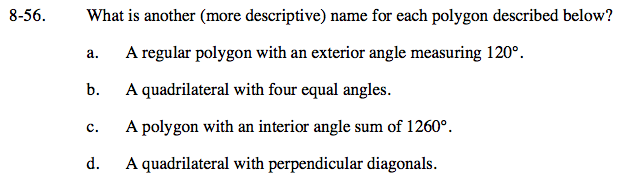Home > GC > Chapter 8 > Lesson 8.1.5 > Problem8-56

8-56.
1. What is another (more descriptive) name for each polygon described below? Homework Help ✎

1. A regular polygon with an exterior angle measuring 120°.

2. A quadrilateral with four equal angles.

3. A polygon with an interior angle sum of 1260°.

4. A quadrilateral with perpendicular diagonals.Review the Math Notes boxes in Lessons 7.1.4 and 8.1.4 for help with regular polygons and interior and exterior angles.

equilateral triangle

rectangle